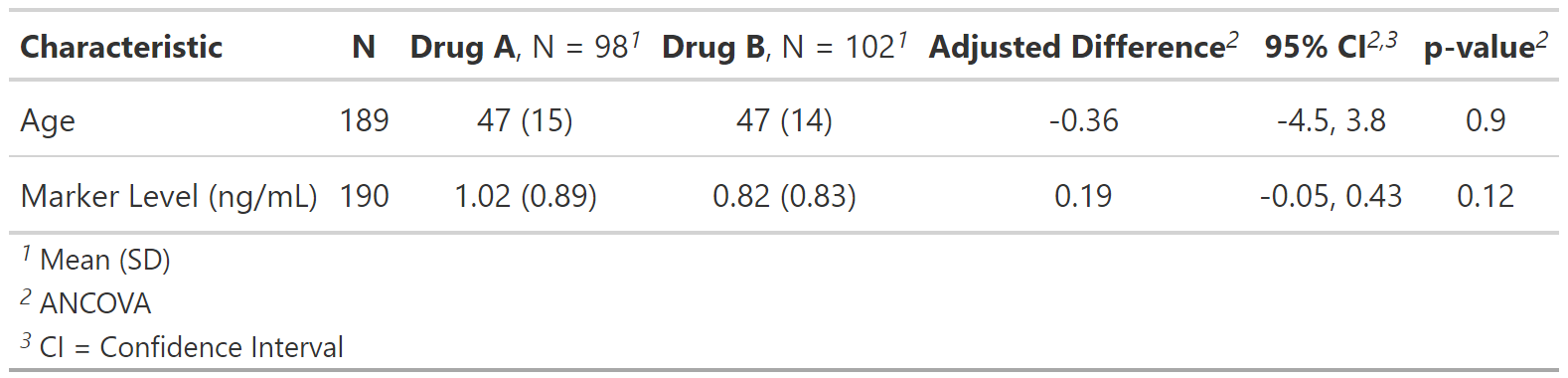Add the difference between two groups (typically mean difference), along with the difference confidence interval and p-value.

add_difference(
x,
test = NULL,
group = NULL,
test.args = NULL,
conf.level = 0.95,
include = everything(),
pvalue_fun = NULL,
estimate_fun = NULL
)

## Arguments

x

"tbl_summary" or "tbl_svysummary" object

test

List of formulas specifying statistical tests to perform for each variable, e.g. list(all_continuous() ~ "t.test"). Common tests include "t.test" or "ancova" for continuous data, and "prop.test" for dichotomous variables. See tests for details and more tests.

group

Column name (unquoted or quoted) of an ID or grouping variable. The column can be used to calculate p-values with correlated data. Default is NULL. See tests for methods that utilize the group= argument.

Variables to include in mean difference adjustment (e.g. in ANCOVA models)

test.args

List of formulas containing additional arguments to pass to tests that accept arguments. For example, add an argument for all t-tests, use test.args = all_tests("t.test") ~ list(var.equal = TRUE)

conf.level

Must be strictly greater than 0 and less than 1. Defaults to 0.95, which corresponds to a 95 percent confidence interval.

include

Variables to include in output. Input may be a vector of quoted variable names, unquoted variable names, or tidyselect select helper functions. Default is everything().

pvalue_fun

Function to round and format p-values. Default is style_pvalue. The function must have a numeric vector input (the numeric, exact p-value), and return a string that is the rounded/formatted p-value (e.g. pvalue_fun = function(x) style_pvalue(x, digits = 2) or equivalently, purrr::partial(style_pvalue, digits = 2)).

estimate_fun

List of formulas specifying the formatting functions to round and format differences. Default is list(all_continuous() ~ style_sigfig, all_categorical() ~ function(x) paste0(style_sigfig(x * 100), "%")) Function to round and format difference. Default is style_sigfig()

## Example Output

Example 1Example 2Review list, formula, and selector syntax used throughout gtsummary

## Examples

# \donttest{
# Example 1 ----------------------------------
trial %>%
select(trt, age, marker, response, death) %>%
tbl_summary(
by = trt,
statistic =
list(
all_continuous() ~ "{mean} ({sd})",
all_dichotomous() ~ "{p}%"
),
missing = "no"
) %>%

# Example 2 ----------------------------------